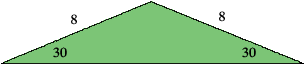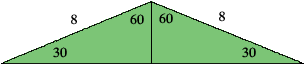SEARCH HOMEMath Central Quandaries & QueriesQuestion from Kim, a student: I am given the length of the two legs of an isosceles triangle (8), and the base angles are 30 degrees...I am asked to find the area of the triangle with only this informationHi Kim,
A picture of our triangle is a good place to start,In Euclidian geometry, the angles of any triangle add up to 180o.

Therefore, the missing angle is 120o.

Now imagine we drew a line which cut the big angle in half.  We can create two identical (why?) triangles.What is the missing angle?
Our new triangles are the so-called “30-60 triangle”, what are the lengths of this ‘special’ triangle’s sides?  What are the lengths of the missing sides for the triangle above?  What is the formula for the area of a triangle?
Hope this helps.
TylerMath Central is supported by the University of Regina and The Pacific Institute for the Mathematical Sciences.